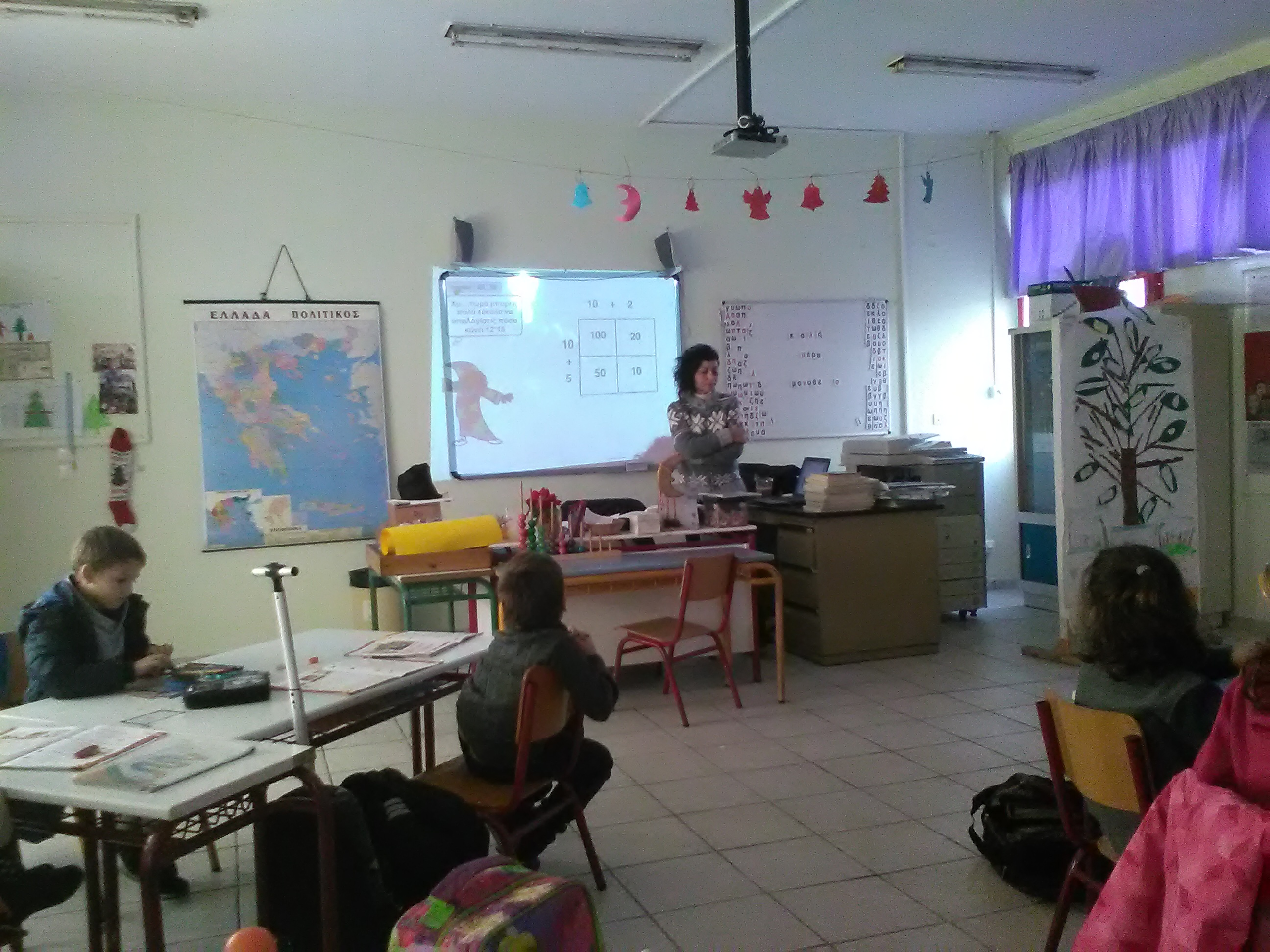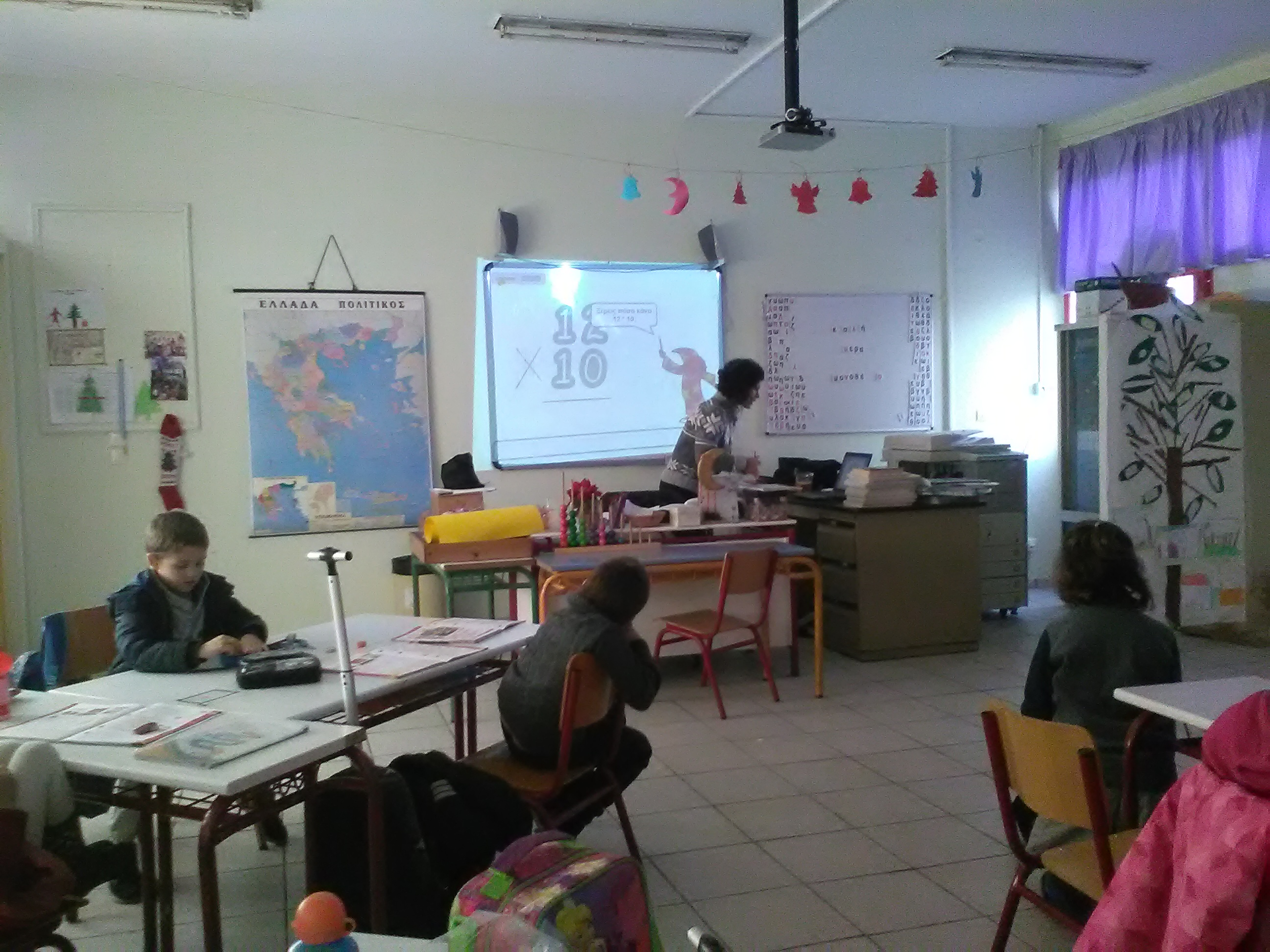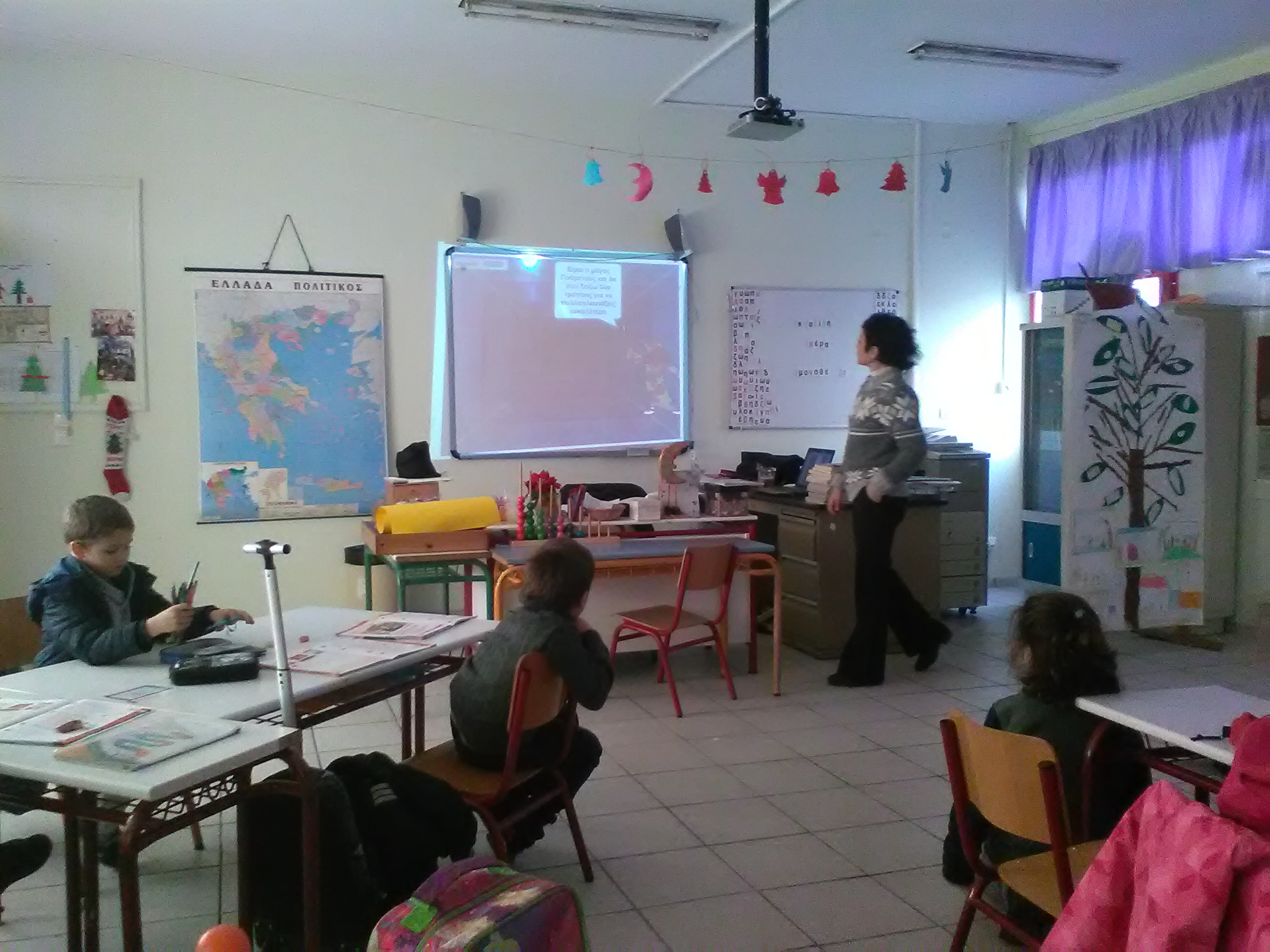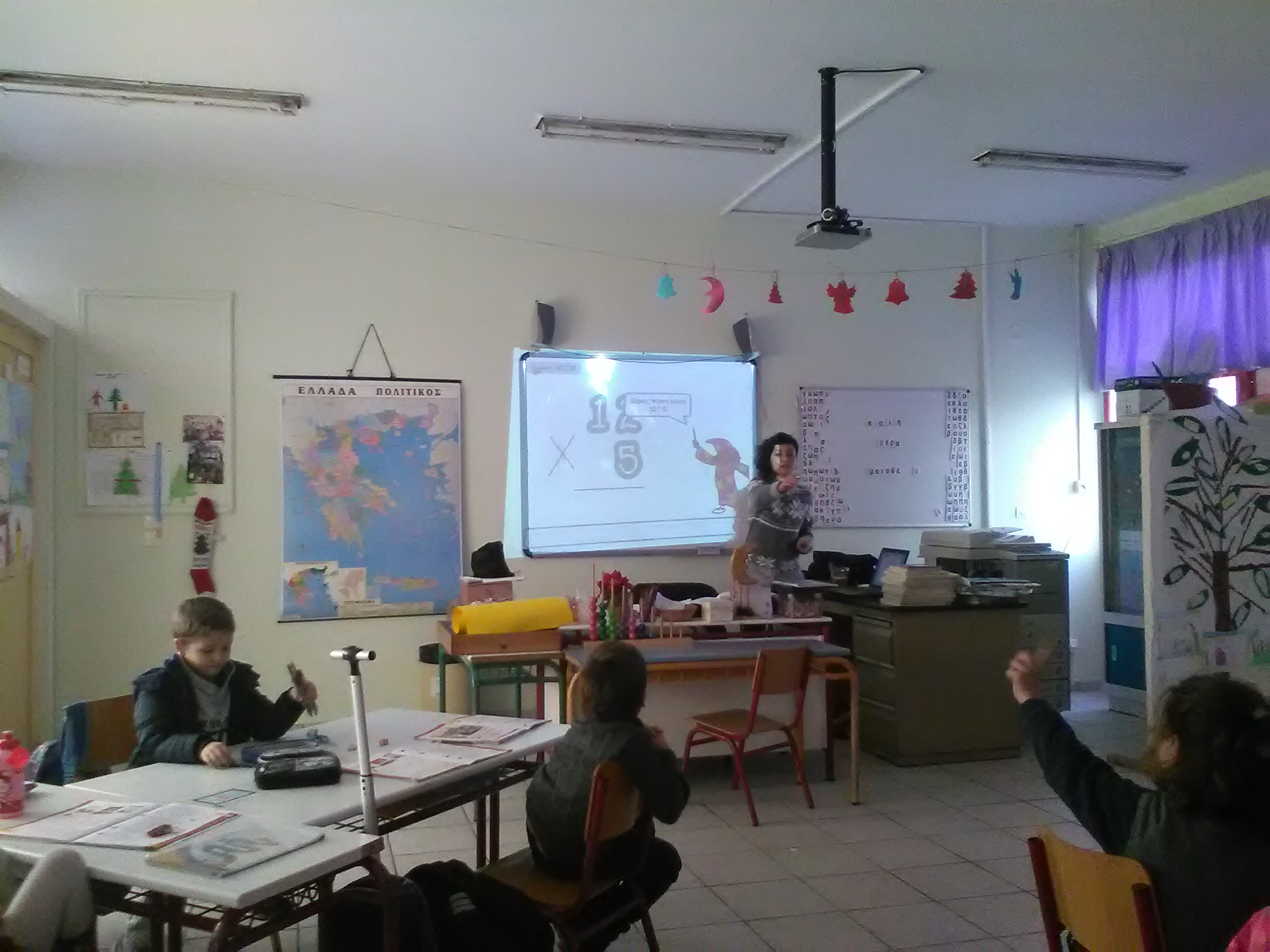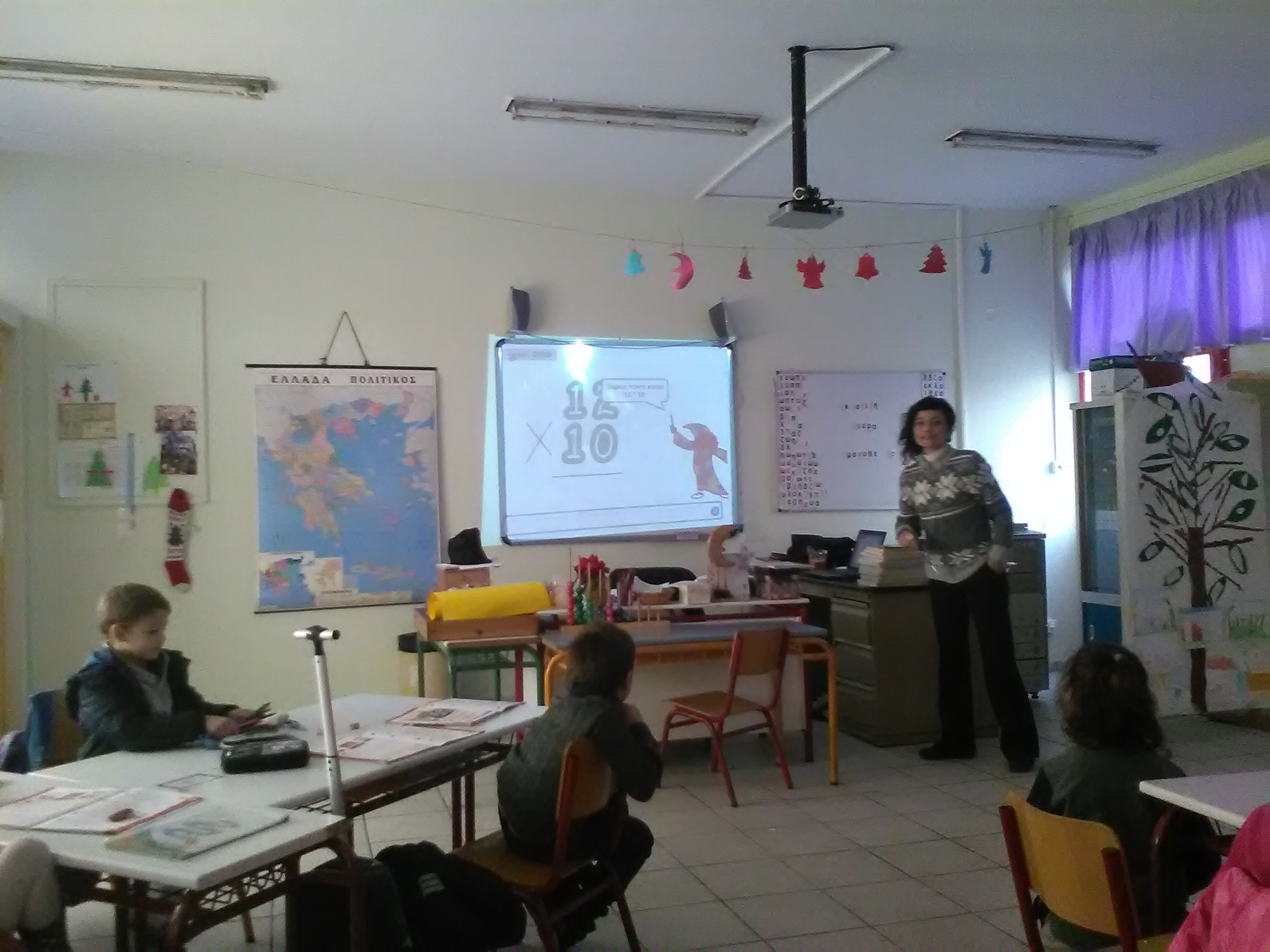##### Ralleia Experimental Primary Schools

Math Lessons with Operations: Multiply in various ways

Teacher: Aikaterini Papasimaki

Class: D (1-class school of Ralleia - Monothesio)

Α. Subject: "Multiply in various ways" Maths 4th Grade

Objectives

1. The scope of this lesson was to teach students to  multiply in different ways.

A1. Rubric for initial assessment

As it was understood from the initial evaluation rubric, students showed an excellent performance levels in knowledge of multiplication table and in calculating the product with the powers of 10. However, there were some small problems as far as the mental multiplications and basic knowledge about the properties of multiplication are concerned. As a result, we decided to conduct a lesson based on scratch in order to eliminate these knowledge gaps.

B. Teaching Maths using Scratch

B.1. First Lesson
The main idea of this course was based on the birth of a magician, the magician Ginomenous (ginomeno in greek means product), who will tour us into the magical world of mathematics starting from the point of quick mental multiplications. Thus, Ginomenous using the calculation of two digit numbers 12 * 15 reveal to students the ‘trickto analyse numbers in units and tens in order to find quickly the product. At the same time, there was a relevant flipchart aimed to help students to understand the process.

Key objectives were
1. Students to perform simple multiplications mentally.
2. Students to develop strategies of calculating products with utilization of commutative and of distributive property in addition.
3. Students initiates to illuminate the distributive property with dual Input panel.

The students seemed to be very interested in the introduction of the teaching of maths by using scratch and much more amused with the presence of their new friend. Then, students having understood the teaching were able to fulfill a worksheet with mental multiplications given to them.

B.2  Second Scratch Lesson

The second lesson was mainly complementary to the first. Specifically, and on the occasion of an educational environmental program for the sea being conducted this year on the 1-class school (Monothesio), I used features of marine mammals at risk in order students to practice quick mental multiplications.

Key objectives were:
1. Students to use strategies for quick mental multiplication with numbers like 9, 19, 29, etc.
2. Students to recognize problem situations in which they can use multiplication.
3. Students to become better acquainted with the distributive property of multiplication as addition and subtraction.

Similarly with the first lesson, students were particularly interested in the new way of teaching. Τhe final cognitive score captured both the educational and the pedagogical value.

C. Rubric for final assessment

Conclusions and remarks from the class teacher

In general it should be stressed that teaching Mathematics by using scratch was a great pedagogical experience for both myself and students. However, there have been some problems mainly as far as my acquaintance with scratch is concerned. Once the problems solved, teaching proceeded normally and it is quite important that students were taught the program in the course of computer science. Furthermore, nowadays the depiction of teaching and the use of new technologies is much closer to the cognitive perception of the students.